## CURVES

 Generate a smoothly interpolated, curved mapping look up table from a set of break points and applies it to an image

 USAGE: curves [-s xscale,yscale] [-g graphmode] "x1,y1 x2,y2 ..." infile outfile USAGE: curves [-s xscale,yscale] [-g graphmode] -f point_file infile outfile USAGE: curves [-h or -help] "x1,y1 x2,y2 ..." ....... break point values of piece-wise linear transformation ......................... enclosed in quotes; minimum of one (x,y) break point pair; ......................... x corresponds to the graylevel in the input image; ......................... y corresponds to the graylevel in the outut image; ......................... x,y non-negative floats enclosed in quotes; ......................... list must be specified just prior to lutfile -c ... channel .......... channel to process; options are: red, green, blue, ......................... lightness, brightness, luma and luminosity; ......................... default=all rgb channels -f ... point_file ....... text file containing list of break points; ......................... one x,y pair per line -s ... xscale,yscale .... range of values for x,y breakpoint pairs from 0 to xscale,yscale; ......................... positive integers; default=100,100 -g ... graphmode ........ enables the creation of a graph of the transformation ......................... and optionally the original break point, which is then ......................... displayed automatically. The choices are: curve (C) or ......................... points (P), where the latter shows the original user ......................... specified break points in addition to the curve. The ......................... default is curve (C). There is a default setting below ......................... that can be changed to enable this to be save to a file ......................... named outfile_graph.gif PURPOSE: To generate a smoothly interpolated, curved mapping look up table from a set of break point and applies it to an image. DESCRIPTION: CURVES takes the supplied break point pairs and generates a dimensional spline curve that is then used to create a 1-D look up table image. The look up table image is then used to change grayscale values in the channels of the input image. At least one point pair must be supplied either as an argument or in a text file with one pair per line supplied. The x value is the position in the look up table which is associated with the grayscale value of the desired channels of the input image and the y value is the associated grayscale value for the corresponding channels in the resulting output image. A Catmull-Rom spline is used to generate the the curve and does go through the supplied point pairs. ARGUMENTS: "x1,y1 x2,y2" ... List of x,y break-points for the curved transformation. The x,y values are non-negative break-point pairs for the curved transformation. There is a minimum one point pair that must be supplied. The x and y values are associated with the input and output grayscale values of the look up table, where x,y values can range from 0 to xscale,yscale. xscale determines the length of the 1D lookup table (length=xscale + 1); that is, the range of x (input) values and yscale determines the range of y (output) values. Both xscale and yscale must be positive integers and their defaults are 100. If the first pair is not 0,0 or the last pair is not xscale,yscale then those points will be added automatically to the list of point pairs. IMPORTANT: the list of break-point pairs must be specified just prior to infile outfile. -f point_file ... point-file is a text file containing the list of break points, one x,y pair per line. -s xscale,yscale ... xscale,yscale is the range of values for the x,y breakpoint pairs which go from 0 to xscale,yscale; positive integers. The default is 100,100. Larger values for xscale and yscale can be used for more accuracy. For example with xscale=yscale=100, x and y values are specified between 0 and 100 and the length of the output image is 101 pixels. For xscale=yscale=255, x and y values are specified # between 0 and 255 and the length of the output image is 256. Equal values for xscale and yscale are not required. -c channel ... CHANNEL to process. Options are: red, green, blue, lightness (L from HSL), brightness (B from HSB), luma (Y from YCbCr) and luminosity (L from Lab). The default=all rgb channels. -g graphmode... enables the creation of a graph of the curved transformation, which is then displayed automatically. The choices are: curve (C) or points (P), where the latter shows the original user specified break points in addition to the curve. The default is no graph if this option is not used. There is also a default setting below that can be changed to enable this to be save to a file named outfile_graph.gif. The graph will be scaled to size 100x100. IMPORTANT: To end the script, close/quit the graph image. REQUIREMENTS: IM 6.7.8.2 or higher when using channel=luminosity. NOTE: Due to changes in IM colorspace, results for versions 6.7.7.8 - 6.8.5.4 will be brighter for channels other than r,g,b CAVEAT: No guarantee that this script will work on all platforms, nor that trapping of inconsistent parameters is complete and foolproof. Use At Your Own Risk.

Thanks to Anthony Thyssen for the technique used to draw the points efficiently

### EXAMPLES

 One-Point Curve (simulates -gamma) Original Dark Image arguments: "25,75" (0,0 25,75 100,100) Transformation Graph (curve only) Transformation Graph (curve with points drawn)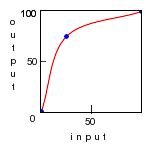S-Curve (simulates -sigmoidal-contrast) Original Image arguments: "0,0 25,15 50,50 75,85 100,100" Transformation Graph (curve only) Transformation Graph (curve with points drawn)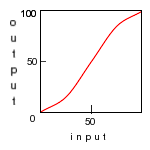Rounded Peak Original Image arguments: "0,0 50,100 100,0" Transformation Graph (curve only) Transformation Graph (curve with points drawn)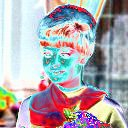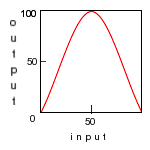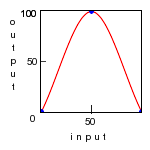Oscillation Original Image arguments: "0,0 25,75 50,50 75,25 100,100" Transformation Graph (curve only) Transformation Graph (curve with points drawn)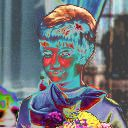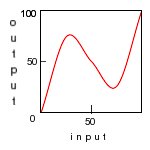What the script does is as follows: Creates a black background image Uses a Catmull-Rom spline to smoothly interpolate between break points Draws a filled white polygon using interpolated break points and additional points needed to make a closed polygon Removes the first row of the image Do a box average of each column in the image to create the lut Apply the lut to the image This is equivalent to the following IM commands. Spline interpolate break points convert \$infile \ \( -size 101x101 xc:black -fill white \ -draw "polygon \$points" \ -crop 101x100+0+1 +repage \ -scale 101x1! \) \ -clut \$outfile